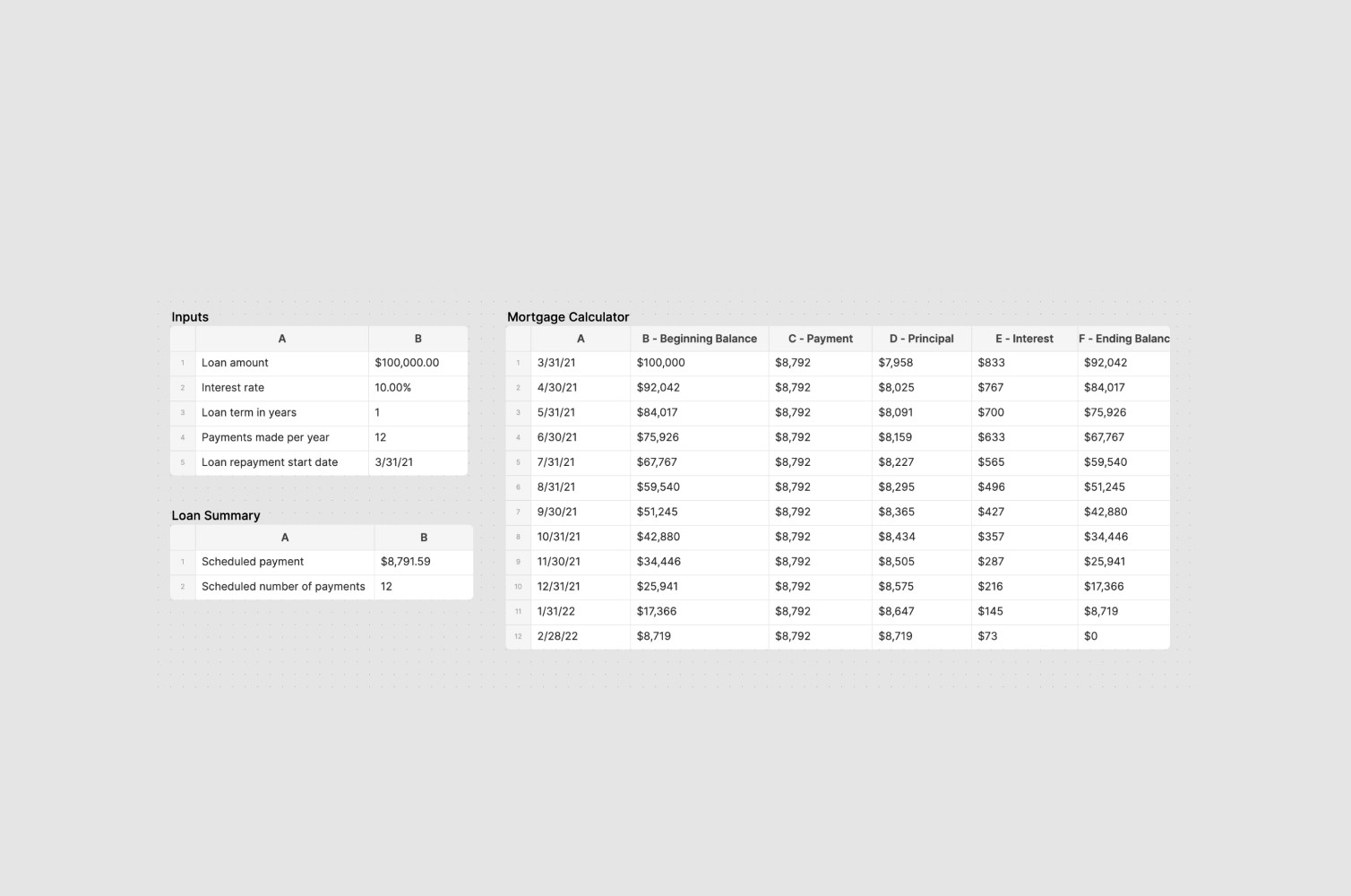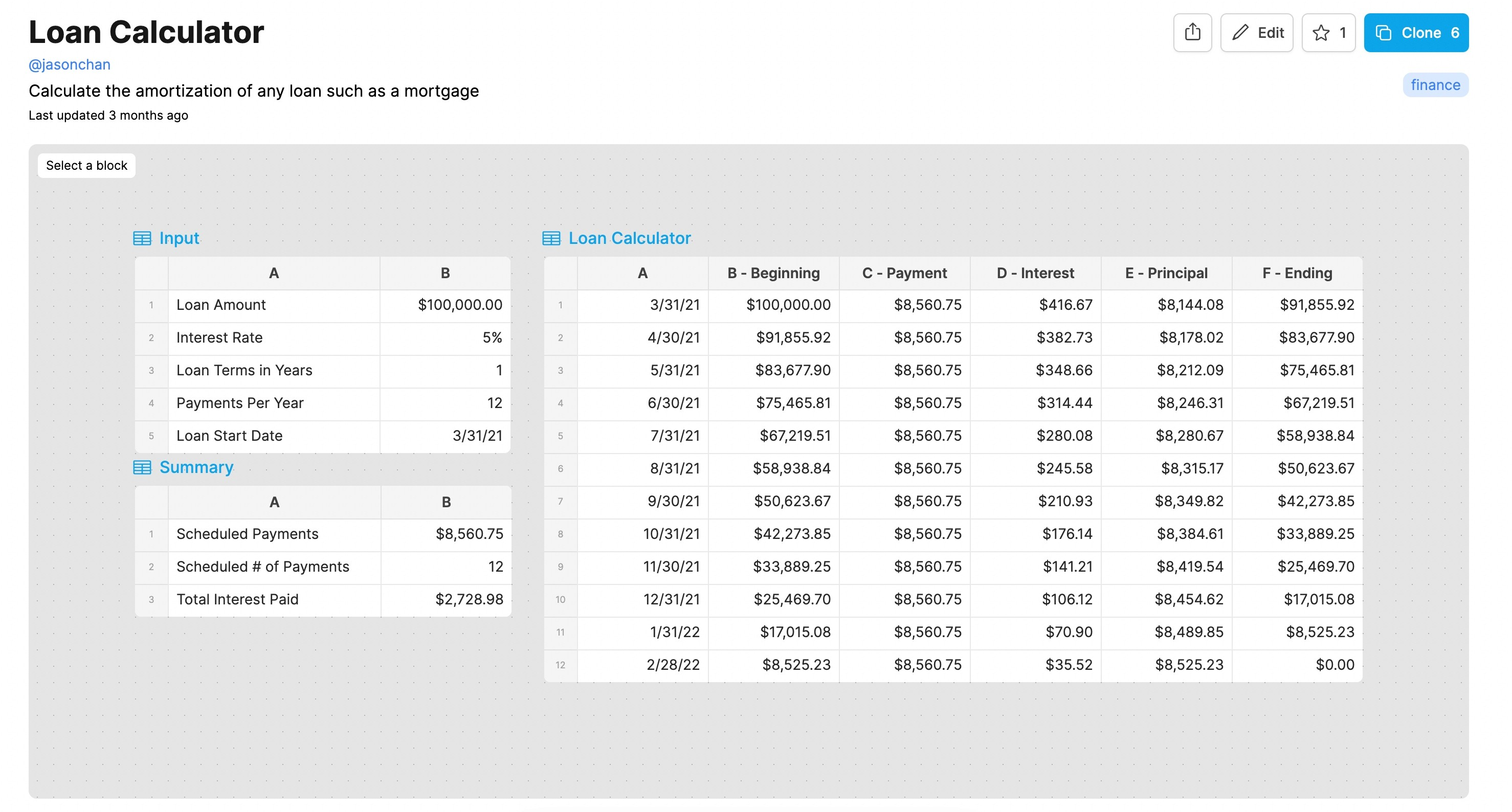# Real Estate Loan Calculator with Amortization (free template)

How to calculate total interest payment for a loan

How to calculate total interest payment for a loan

How to calculate total interest payment for a loan

Aug 31, 2022

Aug 31, 2022Here is the calculator in case you're only looking for the template.

--

Most people buying a home in the US end up taking out some type of loan or a mortgage to help pay for their home. For first time home-buyers, it’s often the largest loan they will take out in their lifetime, so making sure that you understand the numbers is critical.

While there are many online calculators that help you calculate your monthly payments or the total interest amount you will pay, I prefer to calculate these things the old fashioned way: a spreadsheet. This way, I can trace every number to make sure I understand exactly how it was calculated.

You’ll just need a few pieces of information to get started: your loan amount, the interest rate, the loan terms (e.g., 15 year loan or 30 year loan), and the number of payments per year(usually 12 monthly payments a year). With these inputs alone, you can build a pretty accurate model of what your monthly obligations will be.

You can find a pre-built and read-to-use real estate loan calculator here and clone it for your own use, but keep reading if you want to learn how to build one for yourself from scratch.

1. First, figure out the total number of payments, which is just the loan terms multiplied by payments per year. For example, if you have a 30 year loan, then you will have 360 total payments (12*30).

2. Then, you want to figure out what your monthly payment will be. Here, you can use the PMT function to figure that out. In a cell, type the formula: =PMT(Interest Rate/payment per year, scheduled number of payments from step 1, total loan amount).

3. With those two numbers, you can start building your schedule, which should start with the month that you will take out the loan and go on for the number of total payments you will have from step 1.

4. Then for each of these months, create the following columns: Beginning Loan Balance, Payment, Interest, Principal Payment, and Ending Loan Balance.

- The beginning loan balance is always the prior month’s loan balance, except for the first month, which will just be the entire loan balance (since you have not paid anything off yet).

- The Payment will always be what you calculated in step 2.

- To calculate the Interest in a cell you will need to create a formula that takes the beginning loan balance for the month multiplied by the interest rate and divided by the total number of payments per year (which is usually 12 monthly payments a year).

- The Principal is just the payment less the interest.

- The Ending Loan Balance is just the Beginning Loan Balance less the Principal.

5. If you followed all of these steps, you should have built a version of the snippet below.Here is the calculator in case you're only looking for the template.

--

Most people buying a home in the US end up taking out some type of loan or a mortgage to help pay for their home. For first time home-buyers, it’s often the largest loan they will take out in their lifetime, so making sure that you understand the numbers is critical.

While there are many online calculators that help you calculate your monthly payments or the total interest amount you will pay, I prefer to calculate these things the old fashioned way: a spreadsheet. This way, I can trace every number to make sure I understand exactly how it was calculated.

You’ll just need a few pieces of information to get started: your loan amount, the interest rate, the loan terms (e.g., 15 year loan or 30 year loan), and the number of payments per year(usually 12 monthly payments a year). With these inputs alone, you can build a pretty accurate model of what your monthly obligations will be.

You can find a pre-built and read-to-use real estate loan calculator here and clone it for your own use, but keep reading if you want to learn how to build one for yourself from scratch.

1. First, figure out the total number of payments, which is just the loan terms multiplied by payments per year. For example, if you have a 30 year loan, then you will have 360 total payments (12*30).

2. Then, you want to figure out what your monthly payment will be. Here, you can use the PMT function to figure that out. In a cell, type the formula: =PMT(Interest Rate/payment per year, scheduled number of payments from step 1, total loan amount).

3. With those two numbers, you can start building your schedule, which should start with the month that you will take out the loan and go on for the number of total payments you will have from step 1.

4. Then for each of these months, create the following columns: Beginning Loan Balance, Payment, Interest, Principal Payment, and Ending Loan Balance.

- The beginning loan balance is always the prior month’s loan balance, except for the first month, which will just be the entire loan balance (since you have not paid anything off yet).

- The Payment will always be what you calculated in step 2.

- To calculate the Interest in a cell you will need to create a formula that takes the beginning loan balance for the month multiplied by the interest rate and divided by the total number of payments per year (which is usually 12 monthly payments a year).

- The Principal is just the payment less the interest.

- The Ending Loan Balance is just the Beginning Loan Balance less the Principal.

5. If you followed all of these steps, you should have built a version of the snippet below.